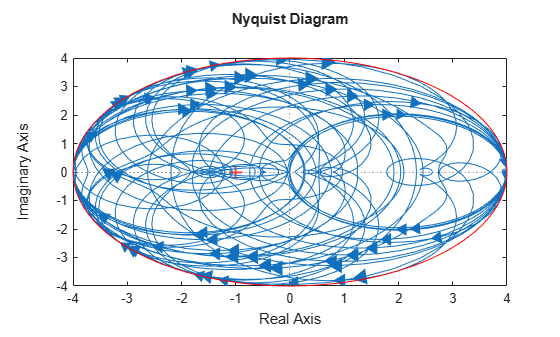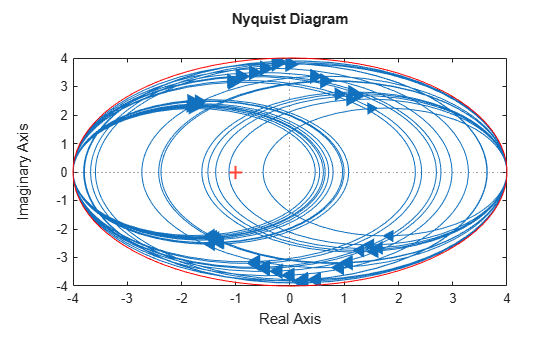Documentation

## Generate Samples of Uncertain Systems

Use the `usample` function to randomly sample an uncertain model, returning one or more non-uncertain instances of the uncertain model.

### Generating One Sample

If `A` is an uncertain object, then `usample(A)` generates a single sample of `A`.

For example, a sample of a `ureal` is a scalar `double`.

```A = ureal('A',6); B = usample(A) B = 5.7298 ```

Create a 1-by-3 `umat` with `A` and an uncertain complex parameter `C`. A single sample of this `umat` is a 1-by-3 double.

```C = ucomplex('C',2+6j); M = [A C A*A]; usample(M) ans = 5.9785 1.4375 + 6.0290i 35.7428 ```

### Generating Many Samples

If `A` is an uncertain object, then `usample(A,N)` generates `N` samples of `A`.

For example, 20 samples of a `ureal` gives a 1-by-1-20 `double` array.

```B = usample(A,20); size(B) ans = 1 1 20 ```

Similarly, 30 samples of the 1-by-3 `umat` `M` yields a 1-by-3-by-30 array.

```size(usample(M,30)) ans = 1 3 30 ```

See Sample Uncertain Elements to Create Arrays for more information on sampling uncertain objects.

### Sampling ultidyn Elements

When sampling an `ultidyn` element or an uncertain object that contains a `ultidyn` element, the result is always a state-space (`ss`) object. The property `SampleStateDimension` of the `ultidyn` class determines the state dimension of the samples.

Create a 1-by-1, gain bounded `ultidyn` object with gain bound 3. Verify that the default state dimension for samples is 1.

```del = ultidyn('del',[1 1],'Bound',3); del.SampleStateDimension```
```ans = 1 ```

Sample the uncertain element at 30 points. Verify that this creates a 30-by-1 `ss` array of 1-input, 1-output, 1-state systems.

```delS = usample(del,30); size(delS)```
```30x1 array of state-space models. Each model has 1 outputs, 1 inputs, and 1 states. ```

Plot the Nyquist plot of these samples and add a disk of radius 3. Note that the gain bound is satisfied and that the Nyquist plots are all circles, indicative of 1st order systems.

```nyquist(delS) hold on; theta = linspace(-pi,pi); plot(del.Bound*exp(sqrt(-1)*theta),'r'); hold off;```Change `SampleStateDimension` to 4, and repeat entire procedure. The Nyquist plots satisfy the gain bound and as expected are more complex than the circles found in the 1st-order sampling.

```del.SampleStateDimension = 4; delS = usample(del,30); nyquist(delS) hold on; theta = linspace(-pi,pi); plot(del.Bound*exp(sqrt(-1)*theta),'r'); hold off;```